## Discuz!应用商城（DisMall）用户名 Email 自动登录 找回密码 密码 立即注册
 搜索 热搜: 活动 交友 discuz

# [求助] 懵逼了，我这数据库好像在默默的查东西

25主题 74积分

Member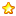0 点

10 个发表于 2021-8-5 14:48:10 | 显示全部楼层 |阅读模式
 2557 Init DB        u3dchina_com                  2556 Query        SELECT `tid` FROM unity_forum_thread WHERE `displayorder` = 0 AND ( `fid` = 43 OR `fid` = 66 ) LIMIT 0,1712                  2556 Query        SELECT COUNT(*) FROM unity_forum_thread WHERE `displayorder` = 0 AND ( `fid` = 43 OR `fid` = 66 )                  2556 Query        SELECT `tid` FROM unity_forum_thread WHERE `displayorder` = 0 AND ( `fid` = 43 OR `fid` = 66 ) LIMIT 0,1712                  2556 Query        SELECT COUNT(*) FROM unity_forum_thread WHERE `displayorder` = 0 AND ( `fid` = 43 OR `fid` = 66 )                  2556 Query        SELECT `tid` FROM unity_forum_thread WHERE `displayorder` = 0 AND ( `fid` = 43 OR `fid` = 66 ) LIMIT 0,1712                  2557 Query        SELECT tid FROM unity_forum_optionvalue4 WHERE 1 AND `archy_qy_3` IN('12','12.1','12.2','12.3','12.4','12.5','12.6','12.7','12.8','12.9','12.10','12.11','12.12','12.13','12.14','12.15','12.16','12.17') AND `archy_zpzw` IN('4') AND `archy_gznx` IN('3') AND `archy_gzdy` IN('5') AND `archy_xlyq` IN('4')  AND fid='48'                  2556 Query        SELECT authorid FROM unity_forum_thread WHERE tid=6839                  2556 Query        SELECT groupid FROM unity_common_member WHERE uid=1                  2556 Query        SELECT authorid FROM unity_forum_thread WHERE tid=6839                  2556 Query        SELECT count(1) FROM unity_forum_post WHERE tid=6839 and authorid = 0                  2557 Query        SELECT COUNT(*) FROM unity_forum_thread t  WHERE t.`fid`='48' AND t.`tid` IN('0') AND t.`displayorder`>='0' AND t.`sortid`='4'                  2557 Query        SELECT * FROM unity_common_cache WHERE `cachekey`='threadclasscount_48'                  2557 Query        SELECT * FROM unity_forum_thread  WHERE `fid`='48' AND `tid` IN('0') AND `displayorder` IN('0','1','2','3','4') AND `sortid`='4'  ORDER BY displayorder DESC, lastpost DESC    LIMIT 20                  2557 Query        SELECT * FROM unity_common_cache WHERE `cachekey`='threadclasscount_48'                  2557 Query        SELECT COUNT(*) FROM unity_forum_thread WHERE `displayorder` = 0 AND ( `fid` = 43 OR `fid` = 66 )                  2557 Query        SELECT `tid` FROM unity_forum_thread WHERE `displayorder` = 0 AND ( `fid` = 43 OR `fid` = 66 ) LIMIT 0,1712                  2556 Quit                         2557 Query        SELECT COUNT(*) FROM unity_forum_thread WHERE `displayorder` = 0 AND ( `fid` = 43 OR `fid` = 66 )                  2557 Query        SELECT `tid` FROM unity_forum_thread WHERE `displayorder` = 0 AND ( `fid` = 43 OR `fid` = 66 ) LIMIT 0,1712                  2557 Query        SELECT COUNT(*) FROM unity_forum_thread WHERE `displayorder` = 0 AND ( `fid` = 43 OR `fid` = 66 )                  2557 Query        SELECT `tid` FROM unity_forum_thread WHERE `displayorder` = 0 AND ( `fid` = 43 OR `fid` = 66 ) LIMIT 0,1712                  2557 Quit                         2558 Connect        u3dchina_com@localhost on                  2558 Query        SET character_set_connection=gbk, character_set_results=gbk, character_set_client=binary,sql_mode=''                  2558 Init DB        u3dchina_com                  2558 Query        SELECT tid FROM unity_forum_optionvalue4 WHERE 1 AND `archy_xlyq` IN('8') AND `archy_zpzw` IN('5') AND `archy_qy_3` IN('32','32.1','32.2','32.3','32.4','32.5','32.6','32.7','32.8','32.9','32.10','32.11','32.12','32.13','32.14') AND `archy_gznx` IN('2') AND `archy_gzdy` IN('4')  AND fid='48'                  2558 Query        SELECT COUNT(*) FROM unity_forum_thread t  WHERE t.`fid`='48' AND t.`tid` IN('0') AND t.`displayorder`>='0' AND t.`sortid`='4'                  2558 Query        SELECT * FROM unity_common_cache WHERE `cachekey`='threadclasscount_48'                  2558 Query        SELECT * FROM unity_forum_thread  WHERE `fid`='48' AND `tid` IN('0') AND `displayorder` IN('0','1','2','3','4') AND `sortid`='4'  ORDER BY displayorder DESC, lastpost DESC    LIMIT 20                  2558 Query        SELECT * FROM unity_common_cache WHERE `cachekey`='threadclasscount_48'                  2558 Query        SELECT COUNT(*) FROM unity_forum_thread WHERE `displayorder` = 0 AND ( `fid` = 43 OR `fid` = 66 )                  2558 Query        SELECT `tid` FROM unity_forum_thread WHERE `displayorder` = 0 AND ( `fid` = 43 OR `fid` = 66 ) LIMIT 0,1712                  2558 Query        SELECT COUNT(*) FROM unity_forum_thread WHERE `displayorder` = 0 AND ( `fid` = 43 OR `fid` = 66 )                  2558 Query        SELECT `tid` FROM unity_forum_thread WHERE `displayorder` = 0 AND ( `fid` = 43 OR `fid` = 66 ) LIMIT 0,1712                  2558 Query        SELECT COUNT(*) FROM unity_forum_thread WHERE `displayorder` = 0 AND ( `fid` = 43 OR `fid` = 66 )                  2558 Query        SELECT `tid` FROM unity_forum_thread WHERE `displayorder` = 0 AND ( `fid` = 43 OR `fid` = 66 ) LIMIT 0,1712                  2558 Quit        然后cpu飙高。。。。。。。。哪个大佬有遇到过吗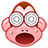25主题 74积分

Member0 点

10 个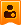楼主| 发表于 2021-8-7 16:19:28 | 显示全部楼层

9主题 1528积分

discuz 老兵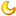0 点

142 个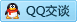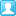发表于 2021-9-6 16:03:17 | 显示全部楼层
 可能是木马 也可能是插件问题

 本版积分规则 回帖后跳转到最后一页

GMT+8, 2022-5-25 15:53 , Processed in 0.058800 second(s), 9 queries , Yac On.